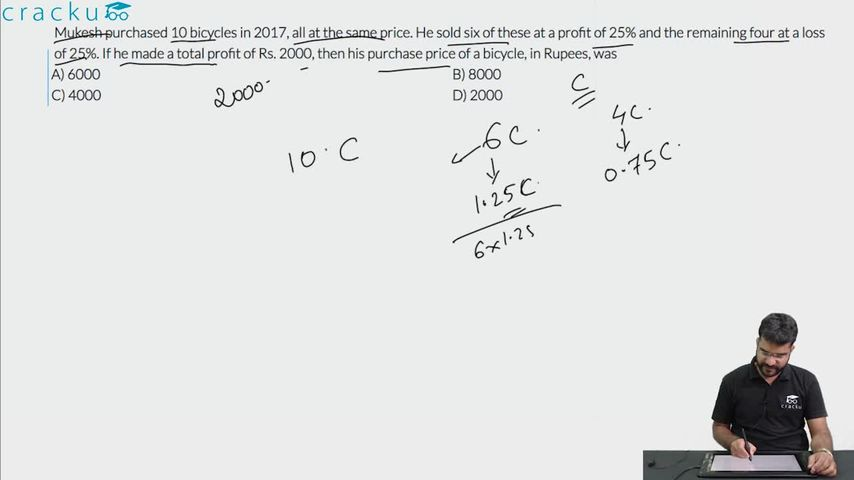Question 28

# Mukesh purchased 10 bicycles in 2017, all at the same price. He sold six of these at a profit of 25% and the remaining four at a loss of 25%. If he made a total profit of Rs. 2000, then his purchase price of a bicycle, in Rupees, was

Solution

Let the cost of each bicycle= 100b

CP of 10 bicycles = 1000b

It is given that he sold six of these at a profit of 25% and the remaining four at a loss of 25%

SP of 10 bicycles = 125b*6+75b*4

=1050b

Profit = 1050b-1000b =50b

50b=2000

CP = 100b = 4000

### View Video Solution